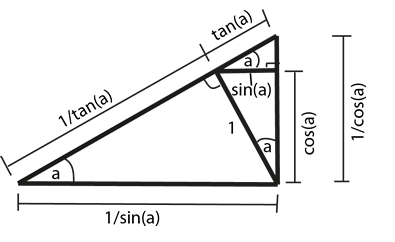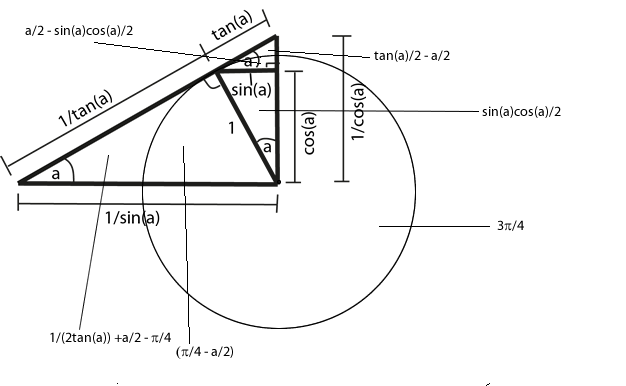#### You may also like### Degree Ceremony

Can you find the sum of the squared sine values?### Logosquares

Ten squares form regular rings either with adjacent or opposite vertices touching. Calculate the inner and outer radii of the rings that surround the squares.### Ball Bearings

If a is the radius of the axle, b the radius of each ball-bearing, and c the radius of the hub, why does the number of ball bearings n determine the ratio c/a? Find a formula for c/a in terms of n.

# Geometric Trig

##### Age 16 to 18 ShortChallenge Level

There are many ways to create the solution as it is something like a jigsaw. We used this method

1. Mark the right angles (use the fact the a radius and tangent at a point are at right angles)
2. Make all occurrences of the angle a
3. Mark the unit length

The diagram then becomesTo work out all of the areas we need to decide what unit of measurement the angle $a$ is in. Of course, we choose radians: there are $2\pi$ radians in a circle. We will also need to know the formula for the area of a circle and the area of a triangle. In this case, the areas are given asThe two largest areas are equal for around 0.40523 radians (23.2 degrees).